# Pair in C++: The Complete Guide

Pair is heterogeneous, i.e., it allows the storage of a variable of different data types. Pair in C++ can be assigned, copied, and compared.

## C++ Pair

C++ Pair is a built-in container in the STL library consisting of two data elements or objects. The pair container is a simple container defined in <utility> header consisting of two data elements or objects.

The first element in the pair is referenced as “first,” and the second element is referenced as “second”.

For accessing the elements, the user can use a variable name followed by a dot operator followed by the keyword first or second.

An array of objects allocated in the map are in pairs by default in which all the ‘first’ elements are unique keys associated with their “second” value objects.

### Syntax

```pair(datatype1, datatype2) Pair_name;
```

### Parameters

1. datatype1, datatype2: It denotes what types of values will be inserted in the pair.
2. Pair_name: The pair’s name will be used for accessing the elements followed by ‘first’ and ‘second.’

Let’s look at the example to make you clear about the syntax.

## C++ Pair Program

Write the program on C++ pair.

```#include <iostream>
#include <utility>

using namespace std;

int main()
{
pair<int, char> PAIR1;
PAIR1.first = 65;
PAIR1.second = 'A';

cout << PAIR1.first << " ";
cout << PAIR1.second << endl;

return 0;
}```

See the output.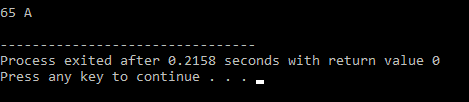Let’s look at another way to initialize values.

### Syntax

```pair (data_type1, data_type2) Pair_name (value1, value2)
```

### Parameters

1. datatype1, datatype2: It denotes what types of values will be inserted in the pair.
2. Pair_name: The pair’s name will be used for accessing the elements followed by “first” and “second”.
3. value1, value2: The values that must be inserted in the pair.

Let’s look at the example to make you clear about the syntax.

## C++ Pair Program

```#include <iostream>
#include <utility>

using namespace std;

int main()
{
pair<string, int> PAIR2("AppDividend", 100);

cout << PAIR2.first << " ";
cout << PAIR2.second << endl;

return 0;
}```

See the output.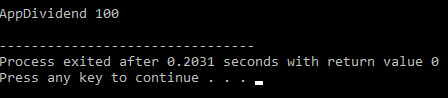See the following program 3.

```#include <iostream>
#include <utility>

using namespace std;

int main()
{
pair<int, double> PAIR1;
pair<string, char> PAIR2;

cout << PAIR1.first;  //it is initialised to 0
cout << PAIR1.second; //it is initialised to 0

cout << " ";

cout << PAIR2.first;  //it prints nothing i.e NULL
cout << PAIR2.second; //it prints nothing i.e NULL

return 0;
}```

See the output.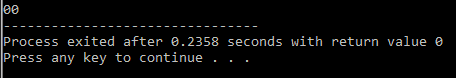If the pair is not initialized with any value, then its first value of the pair will get automatically initialized.

## Different ways to initialize Pair

#### pair p1;

It is a default way of expressing a pair.

#### pair p2(1, ‘a’);

It is initialized with different data types.

#### pair p3(1, 10);

It is initialized with the same data type.

#### pair p4(p3);

It is used for making a copy of p3.

Another way of creating pair is by using the member function make_pair.

## Member Function

### make_pair

SYNTAX:

`Pair_name = make_pair (value1, value2);`

The advantage of using the make_par() function is that it allows making a pair of values without writing the types explicitly.

See the following program.

```#include <iostream>
#include <utility>

using namespace std;

int main()
{
pair<int, char> PAIR1;
pair<string, double> PAIR2("AppDividend", 100);
pair<string, double> PAIR3;

PAIR1.first = 100;
PAIR1.second = 'G';

PAIR3 = make_pair("AppDivided is Best for C++", 4.0);

cout << PAIR1.first << " ";
cout << PAIR1.second << endl;

cout << PAIR2.first << " ";
cout << PAIR2.second << endl;

cout << PAIR3.first << " ";
cout << PAIR3.second << endl;

return 0;
}```

See the output.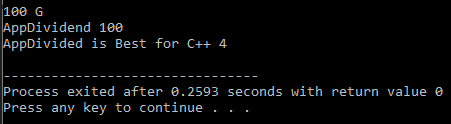Unlike other STL container operators can also be used with a PAIR container.

### Operators

#### Equal Operator (=)

It is used for assigning a new object for a pair object.

SYNTAX:

`pair& operator= (const pair&pr);`

Here, pr is assigned as the new content for the pair object.

#### Comparison operator (==):

It is used for comparing two pairs, i.e., the ‘first’ value of the first pair (Pair1.first) will be compared with the ‘first’ value of the second pair (Pair2.first), and the ‘second’ value of the first pair (Pair1.second) will be compared with ‘second’ value of second pair (Pair2.second).

#### Not Equal Operator (! =):

An equal operator compares the ‘first’ value of two pairs. If both the values get equal, then it will check for the ‘second’ value of both pairs.

#### Logical Operator (>=, <=):

It checks both pairs’ values and returns 0 if it is false and 1 if it is true.

Let’s look at an example to make you clear about all these operators.

Now, let’s see the next program.

```#include <iostream>
#include <utility>

using namespace std;

int main()
{
pair<int, int> pair1 = make_pair(2, 10);
pair<int, int> pair2 = make_pair(9, 10);

cout << (pair1 == pair2) << endl; //comparision operator
cout << (pair1 != pair2) << endl; //not equal operator
cout << (pair1 >= pair2) << endl; //Logical operator
cout << (pair1 <= pair2) << endl; //logical operator
cout << (pair1 > pair2) << endl;  //logical operator
cout << (pair1 < pair2) << endl;  //logical operator

return 0;
}```

See the output.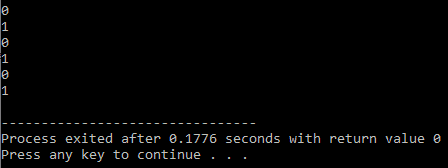### Swap

The swap() function is used for swapping the contents of one pair with another, i.e., pair1.first will be swapped with pair2.first and pair1.second will be swapped with pair2.second.

See the following code example.

```#include <iostream>
#include <utility>

using namespace std;

int main()
{
pair<char, int> pair1 = make_pair('A', 10);
pair<char, int> pair2 = make_pair('B', 20);

cout << "Before swapping:\n ";
cout << "Contents of pair1 = " << pair1.first << " " << pair1.second;
cout << "Contents of pair2 = " << pair2.first << " " << pair2.second;
pair1.swap(pair2);

cout << "After swapping:\n ";
cout << "Contents of pair1 = " << pair1.first << " " << pair1.second;
cout << "Contents of pair2 = " << pair2.first << " " << pair2.second;

return 0;
}```

See the output.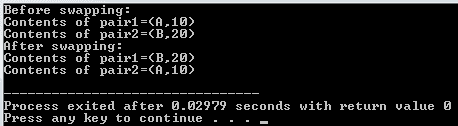## Conclusion

We have discussed Pairs, which are very commonly required in programming competitions, and make sure things are straightforward to implement.

That’s it.

Categories C++

This site uses Akismet to reduce spam. Learn how your comment data is processed.